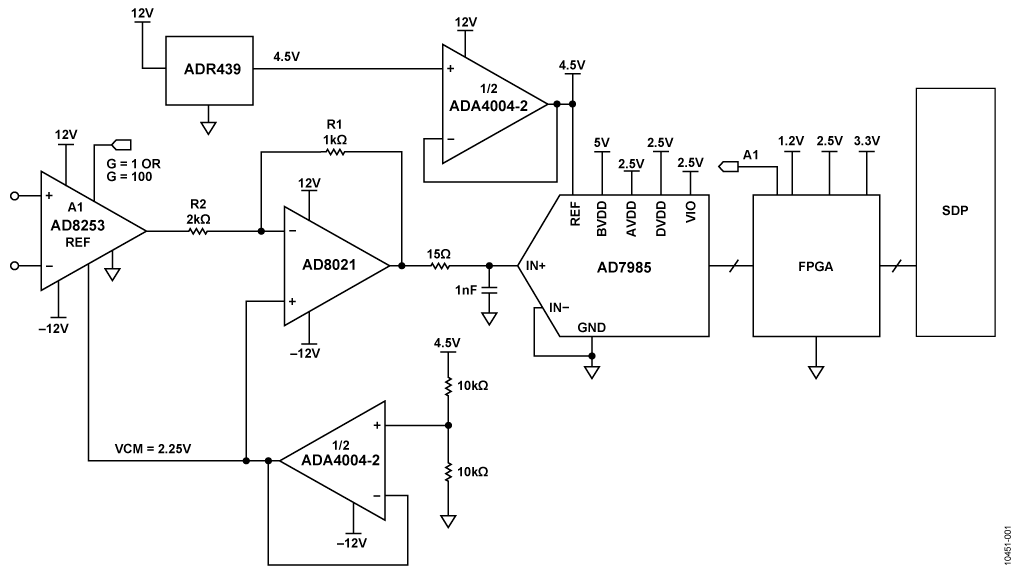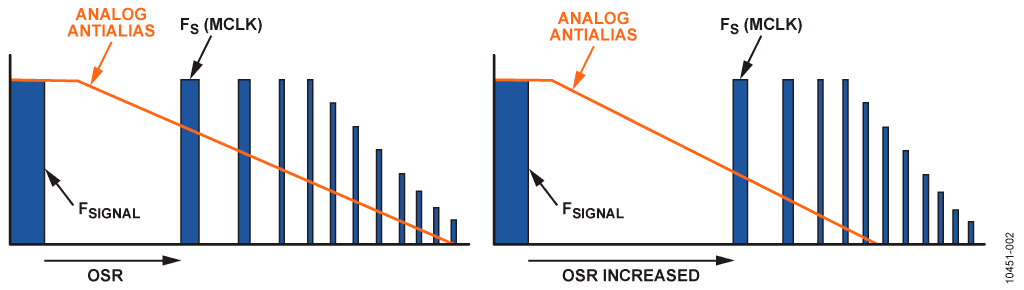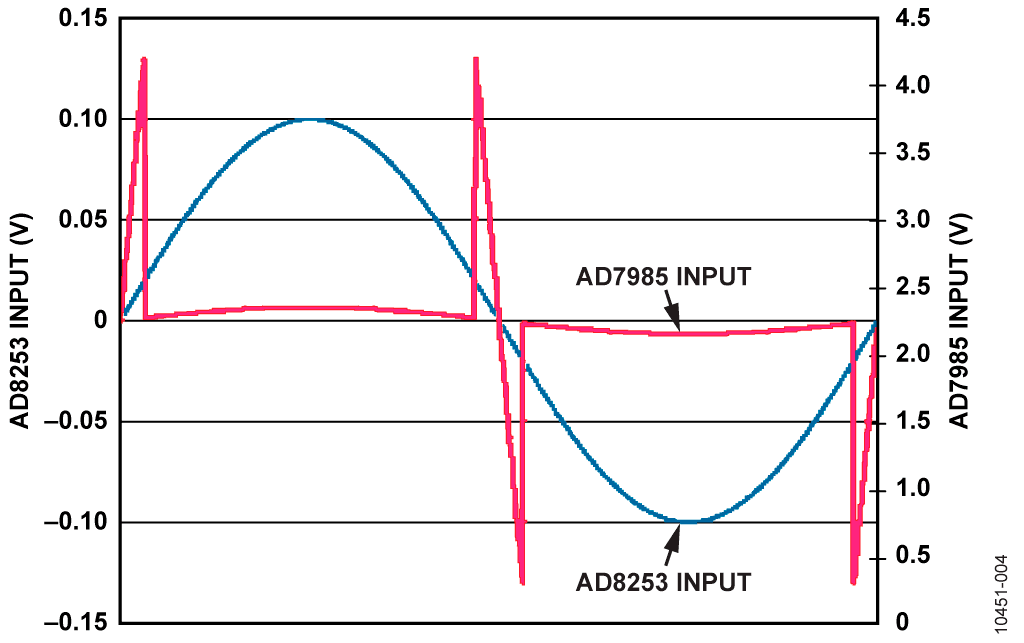## Ресурсы проектирования

### Файлы проектирования и интеграции

• Schematic
• Bill of Materials
• Gerber Files
• Assembly Drawing
Загрузить файлы проектов 1574 kB

## Особенности и преимущества

• Oversampled 16-Bit PulSAR ADC with PGA
• Greater than 125 dB Dynamic Range
• No Pipeline Delay

## Функции и преимущества схемы

This circuit, shown in Figure 1, is a flexible sensor signal conditioning block, with low noise, relatively high gain, and the ability to dynamically change the gain in response to input level changes without affecting performance, while still maintaining a wide dynamic range. Existing sigma-delta technology can provide the dynamic range needed for many applications, but only at the expense of low update rates. This circuit presents an alternative approach that uses the AD7985 16-bit, 2.5 MSPS PulSAR® successive-approximation ADC, combined with an autoranging AD8253 iCMOS® programmable gain instrumentation amplifier (PGA) front end. With gain that changes automatically based on analog input value, it uses oversampling and digital processing to increase the dynamic range of the system to more than 125 dB.Figure 1. Wide Dynamic Range Signal Conditioning Circuit with Autoranging PGA and Oversampling SAR ADC (Note: All Connections and Decoupling Not Shown)## Описание схемы

There are many applications that require wide dynamic range. Weigh scale systems typically use load cell bridge sensors with maximum full-scale outputs of 1 mV to 2 mV. Such systems may require resolutions on the order of 1,000,000 to 1, which, when referred to a 2 mV full-scale input, call for a high performance, low noise, high gain amplifier and a sigma-delta modulator. Similarly, chemical and blood analyses for medical applications often use photodiode sensors, producing very small currents that need to be accurately measured. Some applications, such as vibration monitoring systems, contain both ac and dc information, so the ability to accurately monitor both small and large signals is growing in importance. Sigma-delta ADCs implement this well in many cases but are limited when both ac and dc measurements are needed and fast gain switching is required.

Oversampling is the process of sampling the input signal at a much higher rate than the Nyquist frequency. As a general rule, every doubling of the sampling frequency yields approximately a 3 dB improvement in noise performance within the original signal bandwidth. The oversampling ADC is followed by digital post-processing to remove the noise outside the signal bandwidth, as shown in Figure 2.

To achieve maximum dynamic range, a front-end PGA stage can be added to increase the effective signal-to-noise ratio (SNR) for very small signal inputs. Consider a system dynamic range requirement of >126 dB. First, calculate the minimum rms noise required to achieve this dynamic range. For example, a 3 V input range (6 V p-p) has a 2.12 V full-scale rms value (6/2√2). The maximum allowable system noise is calculated as

126 dB = 20 log (2.12 V/rms noise)

Thus, the rms noise ≈ 1 µV rms.

Now, consider the system update rate, which will determine the oversampling ratio and the maximum amount of noise, referred to the input (RTI), that can be tolerated in the system. For example, with the AD7985 16-bit, 2.5 MSPS PulSAR ADC running at 600 kSPS (11 mW dissipation) and an oversampling ratio of 72, the effective throughput rate of the system after averaging and decimation is 600 kSPS ÷ 72 = 8.33 kSPS. The input signal is therefore limited to a bandwidth of approximately 4 kHz.

The total rms noise is simply the noise density (ND) times √f, so the maximum allowable input spectral noise density (ND) can be calculated as

1 µV rms = ND × √4 kHz

Or, ND = 15.8 nV/√Hz.

From this figure of merit for RTI system input noise, a suitable instrumentation amplifier can be chosen that will provide sufficient analog front-end gain (when summed with the SNR of the ADC, with associated oversampling) to achieve the required 126 dB. For the AD7985, the typical SNR figure is 89 dB, and oversampling by 72 yields another ~18 dB improvement (72 is approximately 26, and each doubling adds 3 dB). Achieving 126 dB DR still requires more than 20 dB improvement, which can come from the gain provided by the analog PGA stage. The instrumentation amplifier must provide a gain of ≥20 (or whatever will not exceed a noise density specification of 15.8 nV/√Hz).

A system-level solution to implement front-end PGA gain and ADC oversampling as discussed above is shown in Figure 1. The input stage uses the AD8253 very low noise 10 nV/√Hz digitally controlled instrumentation amplifier. Gain options are the following: G = 1, 10, 100, 1000.

The AD8021 is a 2.1 nV/√Hz low noise, high speed amplifier capable of driving the AD7985. It also level shifts and attenuates the AD8253 output. Both the AD8253 and AD8021 are operated with an external common-mode bias voltage of 2.25 V, which combine to maintain the same common-mode voltage on the input to the ADC. With a 4.5 V reference, the input range of the ADC is 0 V to 4.5 V.The output of the AD8021 is measured using a high speed ADC. The PGA gain can be dynamically set based on the amplitude of the input signal. For small signal inputs, a gain of 100 is programmed. For larger inputs, the gain is reduced to 1.

Digital post-processing is implemented using the AD7985 16-bit, 2.5 MSPS PulSAR ADC (11 mW dissipation) in a QFN package, which, by virtue of its fast sampling rates, can also be used to implement high orders of oversampling for low input bandwidth applications. Since the complete system noise budget is 15.8 nV/√Hz maximum, referred to the input (RTI), it is useful to calculate the dominant noise sources of each block to ensure that the 15.8 nV/√Hz hard limit is not exceeded. The AD8021 has an input-referred noise specification of <3 nV/√Hz, which is negligible when referred back to the input of the gain-of-100 AD8253 stage. The AD7985 has a specified SNR of 89 dB, using an external 4.5 V reference, for a noise resolution of <45 µV rms.

Considering the Nyquist bandwidth of 300 kHz for the ADC, it will contribute ~83 nV/µHz across this bandwidth. When referred back to the input of the AD7985, its <1 nV/√Hz noisewill be negligible in the system, where the RTI noise sources are summed using a root-sum-of-squares calculation.

A further benefit of using the AD8253 is that it has digital gain control, which allows the system gain to be dynamically changed in response to changes in the input. This is achieved by intelligently using the system’s digital signal processing capability. The main function of digital processing in this application is to produce a higher resolution output, using the AD7985 16-bit conversion results. This is achieved by averaging and decimating the data and switching the analog input gain automatically, depending on the input amplitude. The oversampling process results in a lower output data rate than ADC sample rate, but with a greatly increased dynamic range.

To prototype the digital side of this application, a field-programmable gate array (FPGA) was used as the digital core. To rapidly debug the system, the analog circuitry and the FPGA were consolidated onto a single board using the system demonstration platform (SDP) connector standard to allow easy USB connectivity to the PC, as shown in Figure 3. The SDP is a combination of reusable hardware and software that allows easy control of and data capture from hardware over the most commonly used component interfaces.

This module outputs a new gain setting based on the current gain setting, two raw ADC samples, and some hard-coded threshold figures. Four thresholds are used in the system. The selection of these thresholds is critical to maximizing the analog input range of the system, ensuring the G = 100 mode is used for as much of the signal range as possible, while also preventing the ADC input from being overdriven. Note that this gain block operates on every raw ADC result, not on data that has been normalized. Bearing this in mind, an illustrative example of some thresholds that could be used in a system such as this (assuming a bipolar system with a mid-scale of zero):

T1 (positive lower threshold): +162

(162 codes above mid-scale)

T2 (negative lower threshold): –162

(162 codes below mid-scale)

T3 (positive upper threshold): +32507

(260 codes below positive full-scale)

T4 (negative upper threshold): –32508

(260 codes above negative full-scale)

When in the G = 1 mode, the inner limits, T1 and T2, are used. When an actual ADC result is between T1 and T2, gain is switched to the G = 100 mode. This ensures that the analog input voltage that the ADC sees is maximized as quickly as possible. Then in the G = 100 mode, the outer limits, T3 and T4, are used. If an ADC result is predicted to be above T3 or below T4, the gain is switched to the G = 1 mode to prevent the input of the ADC from being overranged, as shown in Figure 4.Figure 4. The Gain from the Amplifier Input to the Converter Input Is Reduced by 100 When the Analog-to-Digital Converter Input Is Predicted to Be Outside the Threshold Limits (Blue Line: Amplifier Input; Red Line: Converter Input.)

When in the G = 100 mode, if the algorithm predicts that the next ADC sample would be just outside the outer threshold (using a very rudimentary linear prediction), giving an ADC result of +32510, the gain is switched to G = 1, so that, instead of +32510, the next ADC result is +325.

### Performance of the Full System

With fully optimized gain and decimation algorithms, the full system is ready to be tested. Figure 5 shows the system response to a large signal 1 kHz input tone of –0.5 dBFS. When the PGA gain of 100 is factored in, the dynamic range achieved is 127 dB. Similarly, when tested for small signal inputs in Figure 6, with an input tone of 70 Hz @ –46.5 dBFS, up to 129 dB of dynamic range is achieved. The improvement in performance at the smaller input tone is expected, as no active switching of gain ranges occurs during this measurement.Figure 5. Response to Large-Scale 1 kHz Signal Showing 127 dB Dynamic Range

The system’s performance relies on the ability to switch the gain dynamically to handle both small- and large-signal inputs. While sigma-delta technology provides excellent dynamic range, the SAR-based solution offers a way to dynamically change the front-end gain based on the input signal, without compromising system performance. This allows both small-signal and large-signal ac and dc inputs to be measured in real time without waiting for system settling time or incurring large glitches due to delayed gain changing.

## Основные варианты исполнения

Other 16-bit PulSAR ADCs that can be considered for this application are the AD7983 (16-bit @ 1.33 MSPS) and the AD7980 (16-bit @ 1 MSPS). The use of 18-bit PulSAR ADCs is also an option, including the AD7986 (18-bit @ 2 MSPS), AD7984 (18-bit @ 1.33 MSPS), and the AD7982 (18-bit @ 1 MSPS).

## Оценка параметров и тестирование схемы

Figure 3 shows the basic test setup used to evaluate the circuit. A complete schematic and layout of the PCB can be found in the CN-0260 Design Support package found at http://www.analog.com/cn0260-designsupport.

It is important to use a low noise, low distortion signal source for the input, such as an Agilent 33120A, Audio Precision System Two 2322, or equivalent.